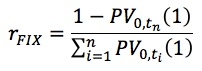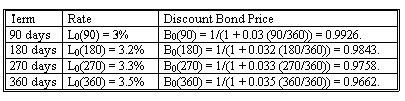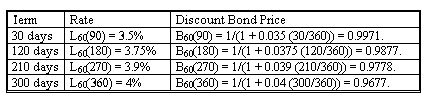### Why should I choose AnalystNotes?

Simply put: AnalystNotes offers the best value and the best product available to help you pass your exams.

##### Subject 10. Equity Swap Contracts
Characteristics:

• One party pays the return on an equity/stock index. The other pays fixed rates, floating rates, or the return on another equity/stock index.
• The rate of return is paid, so the payment can be negative.
• Payment is not determined until the end of the period.

Example 1

IVM enters into a swap with FNS to pay S&P 500 Total Return and receive a fixed rate of 3.45%. The index starts at 2710.55. Payments are made every 90 days for one year. Net payment will be \$25,000,000 [0.0345 x (90/360) - Return on stock index over settlement period].

After-the-fact payments:This combination of outcomes on the above dates represents only one of an infinite number of possible outcomes of the swap. They are used to illustrate how the payments are determined and not the likely results.

• The fixed payment will be \$25,000,000 (.0345) (90/360) = \$215,625.
• The first equity payment is \$25,000,000 (2764.90/2710.55 - 1) = \$501,282.
• So the first net payment is IVM paying \$285,657.

If IVM had received floating, the payoff formula would be (Notional principal) x [LIBOR x (days/360) - Return on stock over settlement period].

If the swap were structured so that IVM pays the return on one stock index and receives the return on another, the payoff formula would be (Notional principal) x [Return on one stock index - Return on the other stock index].

Pricing an Equity Swap

The pricing of an equity swap is identical to the pricing of a comparable interest rate swap.Continue with IVM's swapThe annualized fixed rate would be rFIX = (360/90) [(1 - 0.9662) / (0.9926 + 0.9843 + 0.9758 + 0.9662)] = 0.0345 or 3.45%.

Valuing an Equity Swap

To value the swap at Time t during its life, consider the party paying fixed and receiving equity.

Vt = FBt(C0) - (St/St-) NAE - PV (Par - NAE)

where:

• FBt(C0) denotes the Time t value of a fixed-rate bond initiated with coupon C0 at Time 0.
• St denotes the current equity price.
• St- denotes the equity price observed at the last reset date.
• PV() denotes the present value function from Time t to the swap maturity time.

Suppose we are now 60 days into the life of the IVM swap. The new term structure is as follows:The stock index is at 2739.60. Thus, the value of the swap per \$1 notional principal is (2739.60/2710.55) - 0.9677 - 0.0345 (90/360) (0.9971 + 0.9877 + 0.9778 + 0.9677) = 0.00911854.

This formulation, however, is from the perspective of the party paying the fixed rate and receiving the equity return. To IVM, the value is actually -0.00911854 per \$1 notional principal. Thus, for a notional principal of \$25 million, the value of the swap is \$25,000,000 x (-0.00911854) = -\$227,964.

User Comment
breh In the first table, the last S&P payment should be -884,518 isn't it?